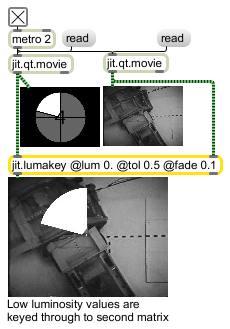# jit.lumakey

Key based on distance from a luminance value

## Description

The jit.lumakey object measures the chromatic distance of each of the left input's cells (pixels) with a reference luminance calulated by the formula
lum = (ascale * alpha_value) + (rscale * r_value) + (gscale * g_value) + (bscale * b_value).

If the distance is less than or equal to a tolerated distance ( tol ) value, the right input cell is multiplied by a maximum keying ( maxkey ) value.

If the distance is greater than the tolerated distance but less than the sum of the tolerated value and a set fade value ( fade ) an interpolated value between the some value between minkey and maxkey values is applied.

For values whose distance is greater than the sum of the tolerated and fade values, the right input cell is multiplied by the minkey value.

The left input is always multiplied by the additive inverse of the left input's multiplicand.

The jit.lumakey object has two modes of output; the first keys between the two input sources, and the second mode will output the keying values (i.e., the left input multiplicands) rather than applying the keying -- the left input is ignored. This is useful for outputting keying information for use with other Jitter objects. Keying can be inverted by swapping the minkey and maxkey values.

## Matrix Operator

matrix inputs:2, matrix outputs:1
 Name IOProc Planelink Typelink Dimlink Plane Dim Type in2 resamp 1 1 1 4 1 char long float32 float64 out n/a 1 1 1 4 1 char long float32 float64

## Attributes

Name Type g/s Description
ascale float The alpha scaling factor (default = 0.)
bscale float The blue scaling factor (default = 0.114)
gscale float The green scaling factor (default = 0.587)
lum float The reference luminance (default = all 0.)
maxkey float The maximum keying (maxkey) value (default = 1.)
minkey float The minimum keying (minkey) value (default = 0.)
mode int Output mode (default = 0 (output keyed matrix))
0 = perform keying and output matrix
1 = output the key map for use elsewhere
2 = alpha contains key map, other planes are from left input
rscale float The red scaling factor (default = 0.299)
tol float The tolerated distance (tol) -- the range of variance from which an input cell's absolute value can diverge when calculating keying (default = 0.)

## Examples